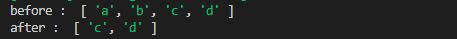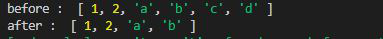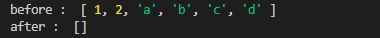# Lodash _.drop() Method

Lodash is a JavaScript library that works on the top of underscore.js. Lodash helps in working with arrays, strings, objects, numbers etc.
The Lodash.drop() method is used to drop the elements in a given array.

Syntax :

`Lodash.drop(array, number)`

Parameters:

• Array: It is the original array from which the elements are to be removed.
• Number: It is the number of elements to be removed from the array.

Note: The elements are removed from the index 0 of the array.

Return Value: It returns the sliced array.

Example 1:

## Javascript

 `// Requiring the lodash library ` `const _ = require(``"lodash"``); ` ` `  `// Original array ` `let array = [``"a"``, ``"b"``, ``"c"``, ``"d"``] ` ` `  `// Ising drop() method to remove ` `// first two elements ` `let newArray = _.drop(array, 2) ` ` `  `// Printing original array  ` `console.log(``"before : "``, array) ` ` `  `// Printing array after applying ` `// drop function ` `console.log(``"after : "``, newArray) `

Output:Example 2: If elements to be removed from the right side of the array we use _.dropRight() function.

## Javascript

 `// Requiring the lodash library ` `let lodash = require(``"lodash"``); ` ` `  `// Original array ` `let array = [1, 2, ``"a"``, ``"b"``, ``"c"``, ``"d"``] ` ` `  `// Using drop() method to remove  ` `// first 2 elements from right ` `let newArray = lodash.dropRight(array, 2) ` ` `  `// Printing original array  ` `console.log(``"before : "``, array) ` ` `  `// Printing array after applying ` `// drop function ` `console.log(``"after : "``, newArray) `

Output:Example 3: If number greater then the size of array is given then it returns the empty array as given in the below given example.

## Javascript

 `// Requiring the lodash library ` `let lodash = require(``"lodash"``); ` ` `  `// Original array ` `let array = [1, 2, ``"a"``, ``"b"``, ``"c"``, ``"d"``] ` ` `  `// Using drop() method to remove ` `// first 10 elements ` `let newArray = lodash.drop(array, 10) ` ` `  `// Printing original array  ` `console.log(``"before : "``, array) ` ` `  `// Printing array after applying ` `// drop function ` `console.log(``"after : "``, newArray) `

Output:My Personal Notes arrow_drop_upCheck out this Author's contributed articles.

If you like GeeksforGeeks and would like to contribute, you can also write an article using contribute.geeksforgeeks.org or mail your article to contribute@geeksforgeeks.org. See your article appearing on the GeeksforGeeks main page and help other Geeks.

Please Improve this article if you find anything incorrect by clicking on the "Improve Article" button below.

Article Tags :

Be the First to upvote.

Please write to us at contribute@geeksforgeeks.org to report any issue with the above content.